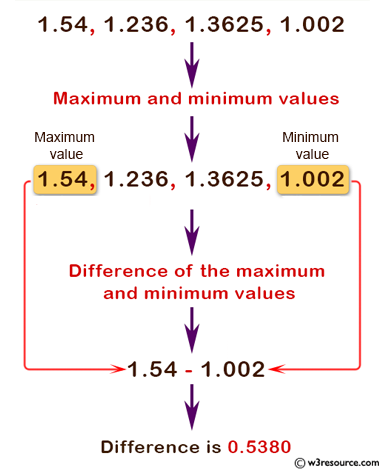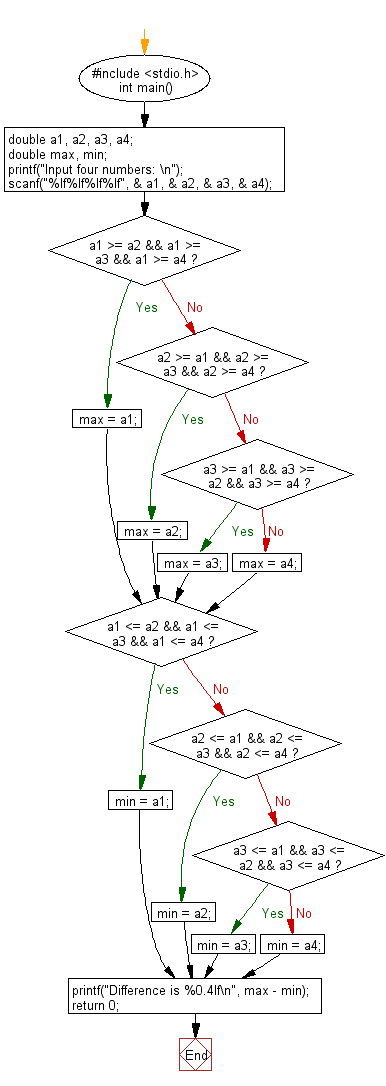﻿ C : Difference between max and min values of 4 numbers

# C Exercises: Print out the difference of the maximum and minimum values of these four numbers

## C Basic Declarations and Expressions: Exercise-58 with Solution

Write a C program that accepts 4 real numbers from the keyboard and prints out the difference between the maximum and minimum values of these four numbers.

Note: Use 4-decimal places.
Test data and expected output:
Input: 1.54 1.236 1.3625 1.002
Output: Difference is 0.5380

Pictorial Presentation:Sample Solution:

C Code:

``````#include <stdio.h>
int main() {
// Declare variables to store four numbers
double a1, a2, a3, a4;
double max, min;

// Prompt user to input four numbers
printf("Input four numbers: \n");
scanf("%lf%lf%lf%lf", &a1, &a2, &a3, &a4);

// Find the maximum among the four numbers
if (a1 >= a2 && a1 >= a3 && a1 >= a4)
max = a1;
else if (a2 >= a1 && a2 >= a3 && a2 >= a4)
max = a2;
else if (a3 >= a1 && a3 >= a2 && a3 >= a4)
max = a3;
else
max = a4;

// Find the minimum among the four numbers
if (a1 <= a2 && a1 <= a3 && a1 <= a4)
min = a1;
else if (a2 <= a1 && a2 <= a3 && a2 <= a4)
min = a2;
else if (a3 <= a1 && a3 <= a2 && a3 <= a4)
min = a3;
else
min = a4;

// Calculate and display the difference between max and min
printf("Difference is %0.4lf\n", max - min);

return 0;
}
``````

Sample Output:

```Input four numbers: 1.54 1.236 1.3625 1.002
Difference is 0.5380
```

Flowchart:C programming Code Editor:

What is the difficulty level of this exercise?

Test your Programming skills with w3resource's quiz.

﻿